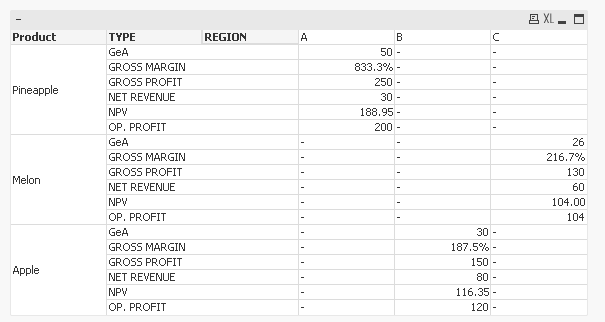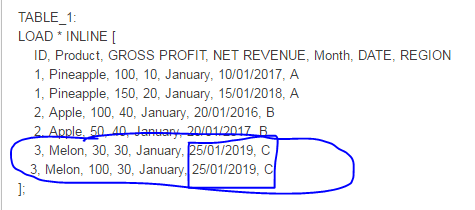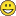New to QlikView

Discussion board where members can get started with QlikView.

Highlighted
Contributor II

Using XNPV function

Hi all,

I have got a table with a expression that calculates the GROSS MARGIN, OPERATIONAL PROFIT and would like to calculate the XNPV for it, but unfortunately it is not accepting set analysis as a parameter.

Please, see below the data model.

TABLE_1:

LOAD * INLINE [

ID, Product, GROSS PROFIT, NET REVENUE, Month, DATE, REGION

1, Pineapple, 100, 10, January, 10/01/2017, A

1, Pineapple, 150, 20, January, 15/01/2018, A

2, Apple, 100, 40, January, 20/01/2016, B

2, Apple, 50, 40, January, 20/01/2017, B

3, Melon, 30, 30, January, 25/01/2019, C

3, Melon, 100, 30, January, 25/01/2019, C

];

Calculation:

'' as [GROSS MARGIN],

'' as [OP. PROFIT],

'' as NPV

Resident TABLE_1;

drop table TABLE_1;

NoConcatenate

TMP:

ID,

Product,

Month,

DATE,

REGION,

[GROSS PROFIT],

[NET REVENUE],

[GROSS MARGIN],

[OP. PROFIT],

NPV

Resident Calculation;

drop table Calculation;

FINAL:

CrossTable(TYPE, REVENUE, 5)

Resident TMP;

drop Table TMP;

For calculating the GROSS MARGIN and OP. PROFIT I follow the instructions from this post Calculating percentages in pivot table

using the expression as below:

=Pick(Match(TYPE, 'GROSS PROFIT', 'NET REVENUE', 'GROSS MARGIN', 'GeA', 'OP. PROFIT'),

Sum(REVENUE),

Sum(REVENUE),

Num(Sum(TOTAL <Product> {<TYPE = {'GROSS PROFIT'}>}REVENUE)/Sum(TOTAL <Product> {<TYPE = {'NET REVENUE'}>}REVENUE), '##.0%'),

Sum(REVENUE)*0.20,

Sum(REVENUE) - Sum(REVENUE)*0.20,

)

The problem is if I try to add XNPV function inside the expression it does not give me the right result, as seen below. In addition, I would like to display the result for each region separate in column. So for dimension it will have PRODUCT and TYPE.

This is only for region A:

=Pick(Match(TYPE, 'GROSS PROFIT', 'NET REVENUE', 'GROSS MARGIN', 'GeA', 'OP. PROFIT', 'NPV'),

Sum( {<REGION={'A'}>} REVENUE),

Sum( {<REGION={'A'}>} REVENUE),

Num(Sum(TOTAL <Product> {<REGION={'A'}, TYPE = {'GROSS PROFIT'}>}REVENUE)/Sum(TOTAL <Product> {<REGION={'A'}, TYPE = {'NET REVENUE'}>}REVENUE), '##.0%'),

Sum( {<REGION={'A'}, TYPE = {'GROSS PROFIT'}>} REVENUE)*0.20,

Sum( {<REGION={'A'}, TYPE = {'GROSS PROFIT'}>} REVENUE) - Sum( {<REGION={'A'}, TYPE = {'GROSS PROFIT'}>} REVENUE)*0.20,

xnpv(0.1, (Sum( {<REGION={'A'}, TYPE = {'GROSS PROFIT'}>} REVENUE) - Sum( {<REGION={'A'}, TYPE = {'GROSS PROFIT'}>} REVENUE)*0.20), DATE)

)

The Desirable result would be like below:

PRODUCTTYPEAB

C

Pineapple

GROSS PROFIT250
PineappleNET REVENUE30
PineappleGROSS MARGIN833%

Pineapple

GeA50
PineappleOP. PROFIT200
PineappleNPV188,94(from excel)

Apple

GROSS PROFIT150
AppleNET REVENUE80
AppleGROSS MARGIN188%
AppleGeA30
AppleOP. PROFIT120
AppleNPV116,36
MelonGROSS PROFIT

130

MelonNET REVENUE60
MelonGROSS MARGIN217%
MelonGeA26
MelonOP. PROFIT104
MelonNPV101,81

Any suggestion on XNPV function?

Thank you!

Tags (7)
10 RepliesMVP

Re: Using XNPV function

The closest I could get was this:Expression:

=Pick(Match(TYPE, 'GROSS PROFIT', 'NET REVENUE', 'GROSS MARGIN', 'GeA', 'OP. PROFIT', 'NPV'),

Sum(REVENUE),

Sum(REVENUE),

Num(Sum(TOTAL <Product> {<TYPE = {'GROSS PROFIT'}>}REVENUE)/Sum(TOTAL <Product> {<TYPE = {'NET REVENUE'}>}REVENUE), '##.0%'),

Sum(TOTAL <Product> {<TYPE = {'GROSS PROFIT'}>} REVENUE)*0.20,

Sum(TOTAL <Product> {<TYPE = {'GROSS PROFIT'}>} REVENUE)*0.80,

Num(XNPV(TOTAL <Product> {<Flag = {1}>}0.10, REVENUE, DATE), '#,##0.00')

)MVP

Re: Using XNPV function

I think melon are off because we have same DATE for both records which could be a typo in the sampleContributor II

Re: Using XNPV function

Thank you Sunny,

The result is what I was looking for, but in my case I have a problem. I cannot flag the 'NEW GROSS PROFIT' field in my code as the value is got by a calculation used in expression. (in the problem case I have put the value straight in table to simplify my question).

Looking at your answer I would like to know if Is there a way of getting the result of last expression,

Sum(TOTAL <Product> {<TYPE = {'GROSS PROFIT'}>} REVENUE)*0.80 and then put in on next expression?

Num(XNPV(TOTAL <Product> (LAST EXPRESSION) 0.10, REVENUE, DATE), '#,##0.00'). Just asking in case we have another possibility of doing that. Otherwise I will have to pass the calculation of GROSS PROFIT to load script and solve the problems I was having.

Thank you.

Contributor II

Re: Using XNPV function

Yes, that's true. Both XNPV and NPV works if we have more than one date at least.MVP

Re: Using XNPV function

We might be able to create an Aggr function here, i just created the flag for simplicity. I don't have access to my computer right now, but will try to get back to you as soon as i get home.

Best,

Sunny

Contributor II

Re: Using XNPV function

Thank you Sunny.

I appreciate your help.

Best Regards.MVP

Re: Using XNPV function

Here you go:

=Pick(Match(TYPE, 'GROSS PROFIT', 'NET REVENUE', 'GROSS MARGIN', 'GeA', 'OP. PROFIT', 'NPV'),

Sum(REVENUE),

Sum(REVENUE),

Num(Sum(TOTAL <Product> {<TYPE = {'GROSS PROFIT'}>}REVENUE)/Sum(TOTAL <Product> {<TYPE = {'NET REVENUE'}>}REVENUE), '##.0%'),

Sum(TOTAL <Product> {<TYPE = {'GROSS PROFIT'}>} REVENUE)*0.20,

Sum(TOTAL <Product> {<TYPE = {'GROSS PROFIT'}>} REVENUE)*0.80,

Num(XNPV(TOTAL <Product> {<TYPE = {'GROSS PROFIT'}>}0.10, REVENUE*0.80, DATE), '#,##0.00')

)

Contributor II

Re: Using XNPV function

I am trying to implement it in my code. That's why I haven't gave you a feedback about that yet. In my example is working perfectly, but in code we have different gross profit for each product and it is obtained only in chart. We don't have the value in load script because when I do that I get an error and due to time I prefer to do everything in a chart.So, one last question for that. Is that possible to do a subtraction of values? Lets take the same example and forget that GeA is 20% from GROSS PROFIT. and OP. PROFIT = GROSS PROFIT - GeA.

In this case I am trying to subtract one NPV per another (I don't know if I will get the right result, doing it to test the possibility), but the second part of expression it is not being recognized. Is there a way of doing that?

Num((XNPV(TOTAL <Product> {<TYPE = {'GROSS PROFIT'}>}0.10, REVENUE, DATE) - XNPV(TOTAL <Product> {<TYPE = {'GeA'}>}0.10, REVENUE*0.2, DATE)), '#,##0.00')

Thank you very much!MVP

Re: Using XNPV function

This seems to be working for me.

=Pick(Match(TYPE, 'GROSS PROFIT', 'NET REVENUE', 'GROSS MARGIN', 'GeA', 'OP. PROFIT', 'NPV'),

Sum(REVENUE),

Sum(REVENUE),

Num(Sum(TOTAL <Product> {<TYPE = {'GROSS PROFIT'}>}REVENUE)/Sum(TOTAL <Product> {<TYPE = {'NET REVENUE'}>}REVENUE), '##.0%'),

Sum(TOTAL <Product> {<TYPE = {'GROSS PROFIT'}>} REVENUE)*0.20,

Sum(TOTAL <Product> {<TYPE = {'GROSS PROFIT'}>} REVENUE)*0.80,

Num((XNPV(TOTAL <Product>{<TYPE = {'GROSS PROFIT'}>} 0.10, REVENUE, DATE) - XNPV(TOTAL <Product> {<TYPE = {'GeA'}>}0.10, REVENUE*0.2, DATE)), '#,##0.00')

)

Set analysis seems to be not recognized by the expression editor, but you still numbers when we click okay. The numbers don't really match your requirement, but they do populate.